##### The Japanese Bonsai specialist
Direct order Contact Help / Services Newsletter# Rankei stone lantern 150 cm

› Lanterns, basins and statues › Stone lantern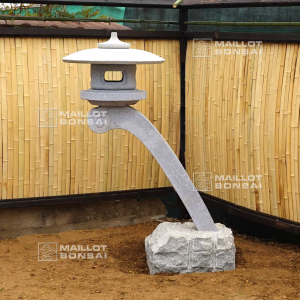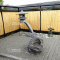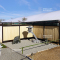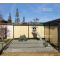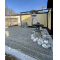ref. : 12006

1.370,00

voluminous/heavy item extra shipping of 75,00

Available quantity : 1Order

###### Description

Japanese garden lantern made of granite.

Height without the base: 150 cm.

Weight: approximately 380 kilograms divided into 6 elements.

Here are the approximate dimensions of each of them: Top element: height 12 cm, diameter 25 cm.

Hat: height 10 cm, diameter 70 cm.

Cage: height 20 cm, diameter 35 cm.

Plate supporting the cage: height 15 cm, diameter 45 cm.

Arch: height 100 cm, section 15*15 cm.

Base: 605020 cm. The lantern is sold alone, without the decoration.

The suggested decoration is an easily achievable staging idea for your space. This style of lantern is not widely known, even in Japan.

It was specially crafted for MAILLOT BONSAI, drawing inspiration from an ancient book on Japanese garden architecture. These models are held in place by a heavy base that serves as a counterweight.

This base, into which the arch fits, will be buried and leveled to ensure proper assembly of all elements.

This model is often placed near bodies of water and rivers. Our garden elements (basins, granite lanterns, stones, and stepping stones) are generally stored outdoors.

Indeed, the patina and aged appearance acquired over time (lichen and moss) add an extra layer of authenticity that is highly valued in traditional Japanese garden art.

#lantern 7.7 #height 6.6 #diameter 4.8 #garden 4.6 #stone 4.5 #base 4.4 #japanese 3.8 #elements 3.8 #this 3.4 #decoration 3

###### Technical description
 Height 100-200 cm

Formule
(( ROUND((CHAR_LENGTH(b.article_nom)-CHAR_LENGTH(REPLACE(b.article_nom, 'height', '')))/LENGTH('height')) + ROUND((CHAR_LENGTH(b.article_description)-CHAR_LENGTH(REPLACE(b.article_description, 'height', '')))/LENGTH('height')) ) * 6.6) + (( ROUND((CHAR_LENGTH(b.article_nom)-CHAR_LENGTH(REPLACE(b.article_nom, 'lantern', '')))/LENGTH('lantern')) + ROUND((CHAR_LENGTH(b.article_description)-CHAR_LENGTH(REPLACE(b.article_description, 'lantern', '')))/LENGTH('lantern')) ) * 5.7) + (( ROUND((CHAR_LENGTH(b.article_nom)-CHAR_LENGTH(REPLACE(b.article_nom, 'diameter', '')))/LENGTH('diameter')) + ROUND((CHAR_LENGTH(b.article_description)-CHAR_LENGTH(REPLACE(b.article_description, 'diameter', '')))/LENGTH('diameter')) ) * 4.8) + (( ROUND((CHAR_LENGTH(b.article_nom)-CHAR_LENGTH(REPLACE(b.article_nom, 'garden', '')))/LENGTH('garden')) + ROUND((CHAR_LENGTH(b.article_description)-CHAR_LENGTH(REPLACE(b.article_description, 'garden', '')))/LENGTH('garden')) ) * 4.6) + (( ROUND((CHAR_LENGTH(b.article_nom)-CHAR_LENGTH(REPLACE(b.article_nom, 'base', '')))/LENGTH('base')) + ROUND((CHAR_LENGTH(b.article_description)-CHAR_LENGTH(REPLACE(b.article_description, 'base', '')))/LENGTH('base')) ) * 4.4) + (( ROUND((CHAR_LENGTH(b.article_nom)-CHAR_LENGTH(REPLACE(b.article_nom, 'japanese', '')))/LENGTH('japanese')) + ROUND((CHAR_LENGTH(b.article_description)-CHAR_LENGTH(REPLACE(b.article_description, 'japanese', '')))/LENGTH('japanese')) ) * 3.8) + (( ROUND((CHAR_LENGTH(b.article_nom)-CHAR_LENGTH(REPLACE(b.article_nom, 'elements', '')))/LENGTH('elements')) + ROUND((CHAR_LENGTH(b.article_description)-CHAR_LENGTH(REPLACE(b.article_description, 'elements', '')))/LENGTH('elements')) ) * 3.8) + (( ROUND((CHAR_LENGTH(b.article_nom)-CHAR_LENGTH(REPLACE(b.article_nom, 'this', '')))/LENGTH('this')) + ROUND((CHAR_LENGTH(b.article_description)-CHAR_LENGTH(REPLACE(b.article_description, 'this', '')))/LENGTH('this')) ) * 3.4) + (( ROUND((CHAR_LENGTH(b.article_nom)-CHAR_LENGTH(REPLACE(b.article_nom, 'decoration', '')))/LENGTH('decoration')) + ROUND((CHAR_LENGTH(b.article_description)-CHAR_LENGTH(REPLACE(b.article_description, 'decoration', '')))/LENGTH('decoration')) ) * 3) + (( ROUND((CHAR_LENGTH(b.article_nom)-CHAR_LENGTH(REPLACE(b.article_nom, 'without', '')))/LENGTH('without')) + ROUND((CHAR_LENGTH(b.article_description)-CHAR_LENGTH(REPLACE(b.article_description, 'without', '')))/LENGTH('without')) ) * 2.7)

## Secure payment## Delivery

Our logistic partners :04 74 55 23 48
Pépinière MAILLOT-BONSAÏ
Le Bois Frazy
01990 RELEVANT - FRANCE
on appointment